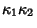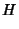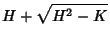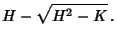## Principal Curvatures

The Maximum and Minimum of the Normal Curvatureandat a given point on a surface are called the principal curvatures. The principal curvatures measure the Maximum and Minimum bending of a Regular Surface at each point. The Gaussian Curvatureand Mean Curvatureare related toandby(1)(2)

This can be written as a Quadratic Equation(3)

which has solutions(4)(5)

See also Gaussian Curvature, Mean Curvature, Normal Curvature, Normal Section, Principal Direction, Principal Radius of Curvature, Rodrigues's Curvature Formula

References

Geometry Center. Principal Curvatures.'' http://www.geom.umn.edu/zoo/diffgeom/surfspace/concepts/curvatures/prin-curv.html.

Gray, A. Normal Curvature.'' §14.2 in Modern Differential Geometry of Curves and Surfaces. Boca Raton, FL: CRC Press, pp. 270-273, 277, and 283, 1993.